# Parallel Processing in Python

Parallel processing can increase the number of tasks done by your program which reduces the overall processing time. These help to handle large scale problems.

In this section we will cover the following topics:

• Introduction to parallel processing
• Multi Processing Python library for parallel processing
• IPython parallel framework

## Introduction to parallel processing

For parallelism, it is important to divide the problem into sub-units that do not depend on other sub-units (or less dependent). A problem where the sub-units are totally independent of other sub-units is called embarrassingly parallel.

For example, An element-wise operation on an array. In this case, the operation needs to aware of the particular element it is handling at the moment.

In another scenario, a problem which is divided into sub-units have to share some data to perform operations. These results in the performance issue because of the communication cost.

There are two main ways to handle parallel programs:

• Shared Memory

In shared memory, the sub-units can communicate with each other through the same memory space. The advantage is that you don’t need to handle the communication explicitly because this approach is sufficient to read or write from the shared memory. But the problem arises when multiple process access and change the same memory location at the same time. This conflict can be avoided using synchronization techniques.

• Distributed memory

In distributed memory, each process is totally separated and has its own memory space. In this scenario, communication is handled explicitly between the processes. Since the communication happens through a network interface, it is costlier compared to shared memory.

Threads are one of the ways to achieve parallelism with shared memory. These are the independent sub-tasks that originate from a process and share memory. Due to Global Interpreter Lock (GIL) , threads can’t be used to increase performance in Python. GIL is a mechanism in which Python interpreter design allow only one Python instruction to run at a time. GIL limitation can be completely avoided by using processes instead of thread. Using processes have few disadvantages such as less efficient inter-process communication than shared memory, but it is more flexible and explicit.

## Multiprocessing for parallel processing

Using the standard multiprocessing module, we can efficiently parallelize simple tasks by creating child processes. This module provides an easy-to-use interface and contains a set of utilities to handle task submission and synchronization.

#### Process and Pool Class

Process

By subclassing multiprocessing.process, you can create a process that runs independently. By extending the `__init__` method you can initialize resource and by implementing `Process.run()` method you can write the code for the subprocess. In the below code, we see how to create a process which prints the assigned id: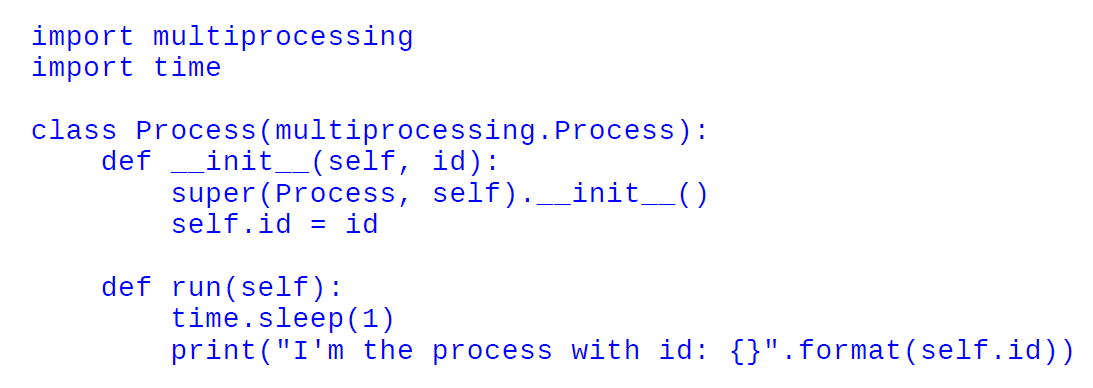To spawn the process, we need to initialize our Process object and invoke `Process.start()` method. Here `Process.start()` will create a new process and will invoke the `Process.run()` method.The code after `p.start()` will be executed immediately before the task completion of process p. To wait for the task completion, you can use `Process.join()`.Here’s the full code:

 `import` `multiprocessing ` `import` `time ` ` `  ` `  `class` `Process(multiprocessing.Process): ` `    ``def` `__init__(``self``, ``id``): ` `        ``super``(Process, ``self``).__init__() ` `        ``self``.``id` `=` `id` `                `  `    ``def` `run(``self``): ` `        ``time.sleep(``1``) ` `        ``print``(``"I'm the process with id: {}"``.``format``(``self``.``id``)) ` ` `  `if` `__name__ ``=``=` `'__main__'``: ` `    ``p ``=` `Process(``0``) ` `    ``p.start() ` `    ``p.join() ` `    ``p ``=` `Process(``1``) ` `    ``p.start() ` `    ``p.join() `

Output: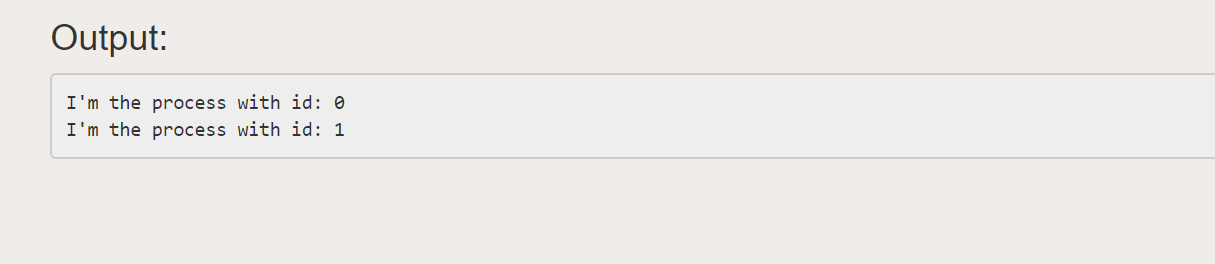Pool class

Pool class can be used for parallel execution of a function for different input data. The `multiprocessing.Pool()` class spawns a set of processes called workers and can submit tasks using the methods `apply/apply_async and map/map_async`. For parallel mapping, you should first initialize a `multiprocessing.Pool()` object. The first argument is the number of workers; if not given, that number will be equal to the number of cores in the system.Let see by an example. In this example, we will see how to pass a function which computes the square of a number. Using `Pool.map()` you can map the function to the list and passing the function and the list of inputs as arguments, as follows: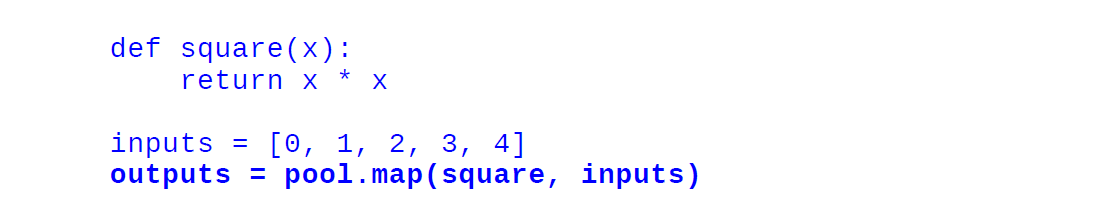`import` `multiprocessing ` `import` `time ` `  `  ` `  `def` `square(x): ` `    ``return` `x ``*` `x ` `  `  `if` `__name__ ``=``=` `'__main__'``: ` `    ``pool ``=` `multiprocessing.Pool() ` `    ``pool ``=` `multiprocessing.Pool(processes``=``4``) ` `    ``inputs ``=` `[``0``,``1``,``2``,``3``,``4``] ` `    ``outputs ``=` `pool.``map``(square, inputs) ` `    ``print``(``"Input: {}"``.``format``(inputs)) ` `    ``print``(``"Output: {}"``.``format``(outputs)) `

Output: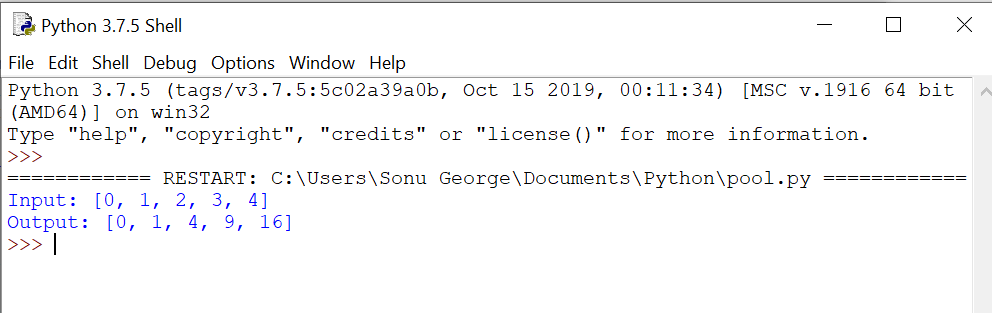When we use the normal map method, the execution of the program is stopped until all the workers completed the task. Using `map_async()`, the AsyncResult object is returned immediately without stopping the main program and the task is done in the background. The result can be retrieved by using the `AsyncResult.get()` method at any time as shown below:`import` `multiprocessing ` `import` `time ` ` `  ` `  `def` `square(x): ` `    ``return` `x ``*` `x ` ` `  `if` `__name__ ``=``=` `'__main__'``: ` `    ``pool ``=` `multiprocessing.Pool() ` `    ``inputs ``=` `[``0``,``1``,``2``,``3``,``4``] ` `    ``outputs_async ``=` `pool.map_async(square, inputs) ` `    ``outputs ``=` `outputs_async.get() ` `    ``print``(``"Output: {}"``.``format``(outputs)) `

Output: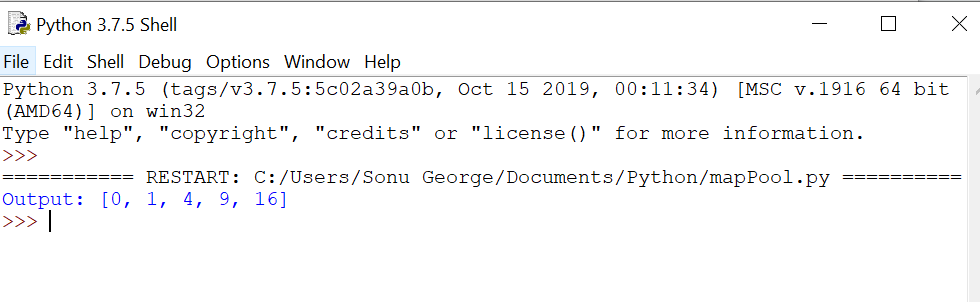`Pool.apply_async` assigns a task consisting of a single function to one of the workers. It takes the function and its arguments and returns an AsyncResult object.`import` `multiprocessing ` `import` `time ` ` `  ` `  `def` `square(x): ` `    ``return` `x ``*` `x ` ` `  ` `  `if` `__name__ ``=``=` `'__main__'``: ` `    ``pool ``=` `multiprocessing.Pool() ` `    ``result_async ``=` `[pool.apply_async(square, args ``=` `(i, )) ``for` `i ``in` `                    ``range``(``10``)] ` `    ``results ``=` `[r.get() ``for` `r ``in` `result_async] ` `    ``print``(``"Output: {}"``.``format``(results)) `

Output: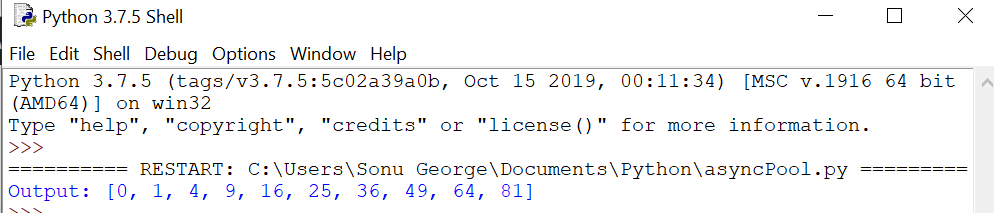## IPython Parallel Framework

IPython parallel package provides a framework to set up and execute a task on single, multi-core machines and multiple nodes connected to a network. In IPython.parallel, you have to start a set of workers called Engines which are managed by the Controller. A controller is an entity that helps in communication between the client and engine. In this approach, the worker processes are started separately, and they will wait for the commands from the client indefinitely.

Ipcluster shell commands are used to start the controller and engines.

`\$ ipcluster start`

After the above process, we can use an IPython shell to perform task in parallel. IPython comes with two basic interfaces:

• Direct Interface

#### Direct Interface

Direct Interface allows you to send commands explicitly to each of the computing units. This is flexible and easy to use. To interact with units, you need to start the engine and then an IPython session in a separate shell. You can establish a connection to the controller by creating a client. In the below code, we import the Client class and create an instance:

```from IPython.parallel import Client
rc = Client()
rc.ids
```

Here, `Client.ids` will give list of integers which give details of available engines.

Using Direct View instance, you can issue commands to the engine. Two ways we can get a direct view instance:

• By indexing the client instance
`dview = rc`
• By calling the DirectView.direct_view method
`dview = rc.direct_view(‘all’).`

As a final step, you can execute commands by using the DirectView.execute method.

`dview.execute(‘ a = 1 ’)`

The above command will be executed individually by each engine. Using the get method you can get the result in the form of an AsyncResult object.

```dview.pull(‘ a ‘).get()
dview.push({‘ a ’ : 2})
```

As shown above, you can retrieve the data by using the `DirectView.pull` method and send the data by using the `DirectView.push` method.

The task-based interface provides a smart way to handle computing tasks. From the user point of view, this has a less flexible interface but it is efficient in load balancing on the engines and can resubmit the failed jobs thereby increasing the performance.

```from IPython.parallel import Client
rc = Client()
```

Using the map and apply method we can run some tasks. In LoadBalanceView the task assignment depends upon how much load is present on an engine at the time. This ensures that all engines work without downtime.

My Personal Notes arrow_drop_upCheck out this Author's contributed articles.

If you like GeeksforGeeks and would like to contribute, you can also write an article using contribute.geeksforgeeks.org or mail your article to contribute@geeksforgeeks.org. See your article appearing on the GeeksforGeeks main page and help other Geeks.

Please Improve this article if you find anything incorrect by clicking on the "Improve Article" button below.

Article Tags :

1

Please write to us at contribute@geeksforgeeks.org to report any issue with the above content.